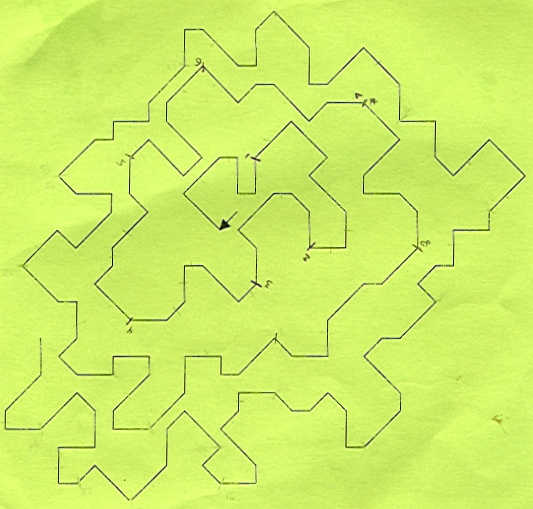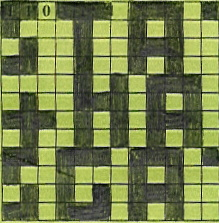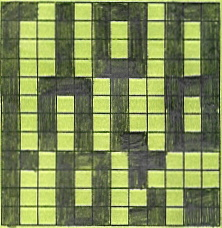CLUE 7- SOLUTION

 Shave and a haircut was a hint to think about "...two bits." The solution was to look at the graph and use it to fill in the two grids (ignore "This is Just a Copy" - that's because we got a second spare set of all of the green sheets, they were marked as copies. We used the originals during the game, so I had to scan in the copies for this page. There are two different grids, which we're supposed to fill in (see below).On the first grid, the first five bits have been filled in: 1 1 0 0 1. If you look at the graph, starting at the arrow, and following the turns, you'll see that the first angle - formed by the arrow with the first line segment - is 90 degrees, the second angle is 90 degrees, the third is not 90 degrees, the fourth is 90 degrees, the fifth is 90 degrees, etc. If you continue the grid, filling in 1's when the angle is 90 degrees and 0's when it is not, you will fill out the grid completely (it's 12 x 12). We filled it out with zeroes and ones but since it's easier to see the solution if the 1 cells are completely blacked out, and the 0 cells are not marked at all, that's what I did here:On the second grid, since we had already used the angles of the lines in the graph, this time we used the lengths. We knew we needed to turn the lengths into some sort of binary arrangement of 1s and 0s, but were confused by the fact that there were four distinct lengths. We called these 1, 2, 3 and 4. There was a pattern to the lengths - the 1's and 3's were always parallel to an edge of the page, and the 2's and 4's were always diagonal to an edge of the page. We called GC for help and they said that we should assign the longer segments (the 3's and 4's) as 1's in the grid, and the shorter segments (the 1's and 2's) as 0's.When the grids were filled in, it became clear that the pattern of 1's spelled something.  If you put the two grids side by side, here's what the final answer looks like.  It helps if you sort of squint at it from a distance with your eyes half-closed. Each line is 4 cells high.STATION + MAIN LOS GATOS. Clue 8